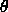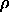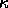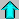# Astronomy 12 - Spring 1999 (S.T. Myers)

## Problem Set #3 (due Thu 18 Feb 1999 5pm)

### Last Revised 23 Mar 1999

Note: If you need help on the Boltzmann and Saha equations, see the online notes for Lecture 9.

1. Consider a photosphere composed of pure neutral hydrogen. At what temperature will the density of atoms in the n=2 excited state and in the n=1 ground state are equal (N_2/N_1 = 1)?

Assume our photosphere has a constant electron pressure of P_e = 2 Pa (2 N/m^2). Use the Saha equation to find the temperature at which the ionized and neutral fractions are equal N_II/N_I = 1.

2. Consider our photosphere of pure hydrogen again. Combine the Boltzmann and Saha equations to estimate the fraction N_2/N of hydrogen atoms in the n=2 state of H I to the total number density of atoms N = N_I + N_II, for stars with temperatures of T=6000 K (type G0), T = 7200 K (F0), T = 10400 K (A0), and T = 27000 K (B0). Again set P_e = 2 Pa. Compare your estimates to Figure 8-13 of the book. Which spectral type should have the strongest Balmer lines, and why?

3. Consider helium in a photosphere of temperature T. The helium atoms will be in ionization equilibrium between neutral He I, singly ionized He II, and doubly ionized He III. Note that helium has a nuclear charge of Z=+2, and the ionization potentials of He I to He II is 24.5 eV, while that from He II to He III is 54.4 eV. Calculate the fractions of the populations in the various ionization states N_II/N_I and N_III/N_II for T = 10000 K, 20000 K, 30000 K and 40000 K. Comment on where the Helium Balmer series strength will be a maximum.

(Hint: assume 2 Pa for the electron pressure, and you will have to put something in for the partition functions, so think carefully about this. If you are inclined, you might go to the 3rd floor DRL library and see if you can find out what the He I,II,III partition functions should be!)

4. According to the standard model of the Sun, the mean opacity in the interior (more correctly, the ``Rosseland Mean Opacity''), has a value of 1.16 cm^2/g (0.116 m^2/kg). The central density for the Sun in this model is 162 g/cm^3 (162000 kg/m^3). Calculate the ``mean free path'' for a photon in the center of the Sun by computing the distance equal to an optical depth of unity. This is how far, on average, a photon will travel before being absorbed.

5. Consider the atmosphere of the Earth as a plane-parallel absorbing medium (ignore emission). On a given night, a particular star, when overhead, has an apparent visual magnitude of m_v = 3. After several hours, the star is at a zenith angle= 60° and has an apparent visual magnitude m_v = 3.1086. Assuming that the atmosphere is uniform and static, calculate the zenith optical depth_0, and the true apparent visual magnitude of the star above the atmosphere. (Hint: Remember that magnitude differences translate into flux or intensity ratios. If you are worrying about solid angles, you should be asking yourself whether the intrinsic angular size of the source enters into the problem at all.)

Assuming that the Earth's atmosphere is 10 km thick, with a temperature of 300 Kelvin, pressure of 1 atm = 10^5 Pa, and is composed of nitrogen N2, calculate the mean density(in kg/m^3) and mean visual opacity(in m^2/kg).Astr12 Index ---Astr12 Home

smyers@nrao.edu Steven T. Myers